Share

# Selina solutions for Concise Physics Class 10 ICSE chapter 4 - Refraction of Light at Plane Surfaces [Latest edition]

Course
Textbook page

#### Chapters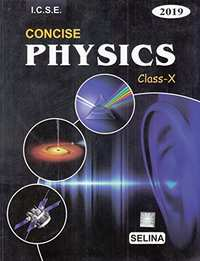## Chapter 4: Refraction of Light at Plane Surfaces

Exercise - 4 (A)Exercise - 4 (B)Exercise - 4 (C)Exercise - 4 (D)

#### Selina solutions for Concise Physics Class 10 ICSE Chapter 4 Refraction of Light at Plane SurfacesExercise Exercise - 4 (A) [Pages 80 - 82]

Exercise - 4 (A) | Q 1 | Page 80

What do you understand by refraction of light?

Exercise - 4 (A) | Q 2.1 | Page 80

Draw diagrams to show the refraction of light from air to glass In  diagram, label the incident ray, refracted ray, the angle of incidence (i) and the angle of refraction (r).

Exercise - 4 (A) | Q 2.2 | Page 80

Draw diagrams to show the refraction of light from glass to air. In  diagram, label the incident ray, refracted ray, the angle of incidence (i) and the angle of refraction (r).

Exercise - 4 (A) | Q 3 | Page 80

A ray of light is incident normally on a plane glass slab. What will be (i) the angle of refraction and (ii) the angle of deviation for the ray?

Exercise - 4 (A) | Q 4 | Page 80

An obliquely incident light ray bends at the surface due to change in speed, when passing from one medium to other. The ray does not bend when it is incident normally. Will the ray have different speed in the other medium?

Exercise - 4 (A) | Q 5 | Page 80

What is the cause of refraction of light when it passes from one medium to another?

Exercise - 4 (A) | Q 6 | Page 80

A light ray suffers reflection and refraction at the boundary in passing from air to water. Draw a neat labelled ray diagram to show it.

Exercise - 4 (A) | Q 7 | Page 80

A ray of light passes from medium 1 to medium 2. Which of the following quantities of the refracted ray will differ from that of the incident ray: Speed, intensity, frequency, wavelength?

Exercise - 4 (A) | Q 8 | Page 81

State the snell’s laws of refraction of light.

Exercise - 4 (A) | Q 9 | Page 81

Define the term refraction index of a medium. Can it be less than 1?

Exercise - 4 (A) | Q 10.1 | Page 81

Compare the speeds of light of wavelength 4000 Å (i.e. violet light) and 8000 Å (i.e. red light) in vacuum.

Exercise - 4 (A) | Q 10.2 | Page 81

How is the refractive index of a medium related to the speed of light in it?

Exercise - 4 (A) | Q 11 | Page 81

A light ray passes from to (a) air, (b) glass. In each case state, how does the speed of light change.

Exercise - 4 (A) | Q 12 | Page 81

A light ray in passing from water to a medium

(a) speeds up

(b) slows down. In each case, give one example of the medium.

Exercise - 4 (A) | Q 13 | Page 81

What do you understand by the statement the refractive index of glass is 1.5 for white light?

Exercise - 4 (A) | Q 14 | Page 81

A monochromatic ray of light passes from air to glass. The wavelength of light in air is λ, the speed of light in air is c and in glass is ν. If the absolute refractive index of glass is 1.5, write down (a) the relationship between 𝒸 and ν, (b) the wavelength of light in glass.

Exercise - 4 (A) | Q 15 | Page 81

In an experiment of finding the refractive index of glass, if blue light is replaced by the red light, how will the refractive index of glass change? Give reason in support of your answer.

Exercise - 4 (A) | Q 16.1 | Page 81

For which colour of white light, is the refractive index of a transparent medium (a) the least (b) the most?

Exercise - 4 (A) | Q 16.2 | Page 81

Which colour of light travels fastest in any medium except air?

Exercise - 4 (A) | Q 17 | Page 81

Name two factors on which the refractive index of a medium depends? State how does it depend on the factors stated by you.

Exercise - 4 (A) | Q 18 | Page 81

How does the refractive index of a medium depend on the wavelength of light used?

Exercise - 4 (A) | Q 19 | Page 81

How does the refractive index of a medium depend on its temperature?

Exercise - 4 (A) | Q 20.1 | Page 81

Light of a single colour is passed through a liquid having a piece of glass suspended in it. On changing the temperature of liquid, at a particular temperature the glass piece is not seen.
When is the glass piece not seen?

Exercise - 4 (A) | Q 20.2 | Page 81

Light of a single colour is passed through a liquid having a piece of glass suspended in it. On changing the temperature of liquid, at a particular temperature, the glass piece is not seen.

Why is the light of a single colour used ?

Exercise - 4 (A) | Q 21 | Page 81

A ray of light from air suffers partial reflection and refraction at the boundary of water.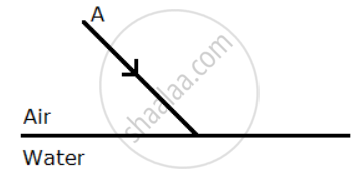Complete the diagram showing (i) the reflected ray B and (ii) the refracted ray C.

How are the angles of incidence i and refraction r related?

Exercise - 4 (A) | Q 22 | Page 81

The diagram alongside shows the refraction of a ray of light from sir to a liquid.
(a) write the values of (i) angle of incidence, (ii) angle of refraction.
(b) use snell’s law to find the refractive index of liquid with respect to air.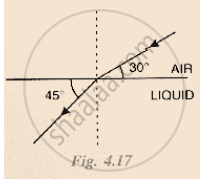Exercise - 4 (A) | Q 23 | Page 81

The refractive index of water with respect to air is a μ w and of glass with respect to air is a μ g.
express the refractive index of glass with respect to water.

Exercise - 4 (A) | Q 24 | Page 81

What is lateral displacement? Draw a ray diagram showing the lateral displacement of a ray of light when it passes through a parallel sided glass slab.

Exercise - 4 (A) | Q 25.1 | Page 81

A ray of light strikes the surface of a rectangular glass block such that the angle of incidence in
air is  0°.
In each case, draw diagram to show the path taken by the ray as it passes through the glass block and emerges from it.

Exercise - 4 (A) | Q 25.2 | Page 81

A ray of light strikes the surface at a rectangular glass slab such that the angle of incidence is 45o.

In each case, draw diagram to show the path taken by the ray as it passes through the glass slab and emerges from it.

Exercise - 4 (A) | Q 26 | Page 81

In the adjacent diagram, AO is a ray of light incident on a rectangular glass block.
(a) complete the path of the ray till it emerges out of the block.
(b) In the diagram, mark the angles of incidence (i) and the angle of refractive index of glass related to the angles 𝔦 and 𝔯 ?
(c) mark angles of emergence by the letter ℯ. How are the angles I and e related?
(d) which two rays are parallel to each other? Name them.
(e) Indicate in the diagram the lateral displacement between the emergent ray and the incident ray.

Exercise - 4 (A) | Q 27 | Page 82

A ray of monochromatic green light enters a liquid from air, as shown in Fig 4.20. The angle 1 is 45° and angle 2 is 30°.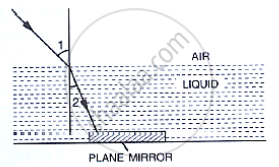(a) Find the refractive index of liquid.
(b) Show in the diagram the path of the ray after it strikes the mirror and re-enters in air. Mark in the diagram the angles where ever necessary.
(c) Redraw the diagram if plane mirror becomes normal to the refracted ray inside the liquid. State the principal used.

Exercise - 4 (A) | Q 28 | Page 82

When an illuminated object is held in front of a thick plane glass mirror, several images are seen, out of which the second image is the brightest. Give reason.

Exercise - 4 (A) | Q 29.1 | Page 82

Fill in the blanks to complete the following sentences:

When light travels from a rarer to a denser medium, its speed. ……………..

Exercise - 4 (A) | Q 29.2 | Page 82

Fill in the blanks to complete the following sentence

When light travels from a denser to a rarer medium, its speed ……………….

Exercise - 4 (A) | Q 29.3 | Page 82

Fill in the blanks to complete the following sentence

The refractive index of glass with respect to air is 3/2. The refractive index of air with respect to glass will be ……………….

#### Selina solutions for Concise Physics Class 10 ICSE Chapter 4 Refraction of Light at Plane SurfacesExercise Exercise - 4 (A) [Page 82]

Exercise - 4 (A) | Q 1 | Page 82

When a ray of light from air enters a denser medium, it:

• Bends away from the normal

• Bends towards the normal

• Goes undeviated

• Is reflected back

Exercise - 4 (A) | Q 2 | Page 82

A light ray does not bend at the boundary in passing from one medium to the other medium if the angle of incident is:

•  45°

•  60°

• 90°

Exercise - 4 (A) | Q 3 | Page 82

The highest refractive index is of :

• Glass

• Water

• Diamond

• Ruby

#### Selina solutions for Concise Physics Class 10 ICSE Chapter 4 Refraction of Light at Plane SurfacesExercise Exercise - 4 (A) [Page 82]

Exercise - 4 (A) | Q 1 | Page 82

The speed of light in air is 3 × 108 m s-1. Calculate the speed of light in glass. The refractive index of glass is 1.5

Exercise - 4 (A) | Q 2 | Page 82

The speed of lllight in diamond is 125,000 km s-1. What is its refractive index? (speed of light in air = 3 × 108 m s-1).

Exercise - 4 (A) | Q 3 | Page 82

The refractive index of water with respect to air is 4/3. What is the refractive index of air with respect to water?

Exercise - 4 (A) | Q 4 | Page 82

A ray of light of wavelength 5400 Å suffer refraction from air to glass. Taking αμℊ = 3/2, find the wavelength of light in glass.

#### Selina solutions for Concise Physics Class 10 ICSE Chapter 4 Refraction of Light at Plane SurfacesExercise Exercise - 4 (B) [Pages 87 - 88]

Exercise - 4 (B) | Q 1 | Page 87

What is a prism?
With the help of diagram of a prism, indicate its refracting surfaces, refracting edge and base.

Exercise - 4 (B) | Q 2 | Page 87

The following diagrams (a) and (b) in Fig. 4.29 below show the refraction of a monochromatic ray of light through a parallel sided glass block and a prism respectively.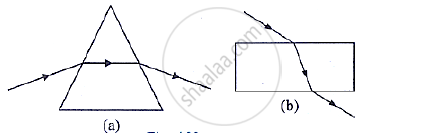(a) In each diagram, label the incident, refracted, emergent rays and the angle of deviation.

(b) In what way the direction of emergent ray in the two cases differ with respect to the incident ray ? Explain your answer.

Exercise - 4 (B) | Q 3 | Page 87

Define the term angle of deviation

Exercise - 4 (B) | Q 4 | Page 87

Complete the following sentence:

Angle of deviation is the angle which the …………………. Ray makes with the direction of ………………….. ray.

Exercise - 4 (B) | Q 5 | Page 87

What do you understand by the deviation produced by a prism? Why is it caused? State three factors on which the angle of deviation depends.

Exercise - 4 (B) | Q 6.1 | Page 87

How does the angle of deviation produced by a prism change with increase in the angle of incidence. Draw a curve showing the variation in the angle of deviation with the angle of incidence at a prism surface.

Exercise - 4 (B) | Q 6.2 | Page 87

Using the curve in part (a) above, how do you infer that for given prism, the angle of minimum deviation δmin is unique for the given light.

Exercise - 4 (B) | Q 7 | Page 87

State whether the following statement is ‘true’ or ‘false’

The deviation produced by a prism is independent of the angle of incidence and is same for all the colours of light .

• True

• False

Exercise - 4 (B) | Q 8.1 | Page 87

How does the deviation produced by a prism depend on the refraction index of its material

Exercise - 4 (B) | Q 8.2 | Page 87

How does the deviation produced by a prism depend on the wavelength of incident light

Exercise - 4 (B) | Q 9.1 | Page 87

How does the angle of minimum deviation produces by a prism change with increase in :

the wavelength of incident light

Exercise - 4 (B) | Q 9.2 | Page 87

How does the angle of minimum deviation produces by a prism change with increase in :

the refracting angle of prism?

Exercise - 4 (B) | Q 10 | Page 87

Write a relation for the angle of deviation (δ) for a ray of light passing through an equilateral prism in terms of the angle of incidence (i) angle of emergence (ℯ) and angle of prism(A).

Exercise - 4 (B) | Q 11 | Page 87

A ray of light incident at an angle of incidence i1 passes through an equilateral glass prism such that the refracted ray inside the prism is parallel to its base and emerges at an angle of emergence i2. (i) How is the angle of emergence ‘i2’ related to the angle of incidence ‘i2’. (ii) what can you say about the angle of deviation in such a situation?

Exercise - 4 (B) | Q 12 | Page 87

Draw a ray diagram to show the refraction of a monochromatic ray through a prism when it suffers minimum deviation. How is the angle of emergence related to the angle of incidence in this position.

Exercise - 4 (B) | Q 13 | Page 87

A light ray of yellow colour is incident on an equilateral glass prism at an angel of incidence equal to 48° and suffers minimum deviation by an angle of 36°. (i) What will be the angle of emergence? (ii) If the angle of incidence is changes to (a) 30°, (b) 60°, state whether the angle of deviation will be equal to, less than or more than 36° ?

Exercise - 4 (B) | Q 14 | Page 87

Name the colour of white light which is deviated (i) the most, (ii) the least, on passing through a prism.

Exercise - 4 (B) | Q 15 | Page 87

Which of the two prism, A made of crown glass and B made of flint glass, deviates a ray of light more?

Exercise - 4 (B) | Q 16 | Page 87

How does the angle of deviation depend on the refracting angle of the prism?

Exercise - 4 (B) | Q 17 | Page 87

An object is viewed through a glass prism with its vertex pointing upwards. It appears to be displaced upward. Explain the reason.

Exercise - 4 (B) | Q 18.1 | Page 88

A ray of light is normally incident on one face of an equilateral glass prism. Answer the following:

What is the angle of incidence on the first face of the prism?

Exercise - 4 (B) | Q 18.2 | Page 88

A ray of light is normally incident on one face of an equilateral glass prism. Answer the following:

What is the angle of refraction from the first face of the prism?

Exercise - 4 (B) | Q 18.3 | Page 88

A ray of light is normally incident on one face of an equilateral glass prism. Answer the following:

what will be the angle of incidence at the second face of the prism?

Exercise - 4 (B) | Q 18.4 | Page 88

A ray of light is normally incident on one face of an equilateral glass prism. Answer the following:

will the light ray suffer minimum deviation by the prism?

Exercise - 4 (B) | Q 19 | Page 88

In following figure  shows two identical prisms A and B placed with their faces parallel to each other A ray of light of single colour PQ is incident at the face of the prism A. complete the diagram to show the path of the ray till it emerges out of the prism B.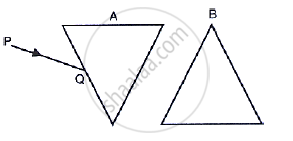[Hint: The e mergent ray out of the prism B will be parallel to the incident ray PQ]

#### Selina solutions for Concise Physics Class 10 ICSE Chapter 4 Refraction of Light at Plane SurfacesExercise Exercise - 4 (B) [Page 88]

Exercise - 4 (B) | Q 1 | Page 88

In refraction of light through a prism, the light ray:

• Suffers refraction only at one face of the prism

• Emerges out from the prism in a direction parallel to the incident ray

• Bends at both the surfaces of prism towards its base

• Bends at both the surfaces of prism opposite to its base.

Exercise - 4 (B) | Q 2 | Page 88

A ray of light suffers refraction through an equilateral prism. The deviation produced by the prism does not depend on the:

• Angle of incidence

• Colour of light

• Material of prism

• Size of prism

#### Selina solutions for Concise Physics Class 10 ICSE Chapter 4 Refraction of Light at Plane SurfacesExercise Exercise - 4 (B) [Page 88]

Exercise - 4 (B) | Q 1 | Page 88

A ray of light incident at an angle 48o on a prism of refracting angle 60o suffers minimum deviation. Calculate the angle of minimum deviation.

[Hint: δmin = 2i - A]

Exercise - 4 (B) | Q 2 | Page 88

What should be the angle of incidence for a ray of light which suffers a minimum deviation of 36o through an equilateral prism?

[Hint: A = 60°, i = (A + δmin)/2]

#### Selina solutions for Concise Physics Class 10 ICSE Chapter 4 Refraction of Light at Plane SurfacesExercise Exercise - 4 (C) [Page 91]

Exercise - 4 (C) | Q 1 | Page 91

How is the refractive index of a medium related to the real and apparent depths of an object in that medium?

Exercise - 4 (C) | Q 2 | Page 91

Prove that

Refractive index ="𝑅𝑒𝑎𝑙 𝑑𝑒𝑝𝑡ℎ "/"𝐴𝑝𝑝𝑎𝑟𝑒𝑛𝑡 𝑑𝑒𝑝𝑡ℎ"

Exercise - 4 (C) | Q 3 | Page 91

A tank of water is viewed normally from above. State how does the depth of tank appear to change. Draw a labelled ray diagram to explain your answer.

Exercise - 4 (C) | Q 4 | Page 91

Water in a pond appears to be only three – quarters of its actual depth. What property of light is responsible for this observation? Illustrate your answer with the help of a ray diagram. How is the refractive index of water calculated from its real and apparent depth?

Exercise - 4 (C) | Q 5 | Page 91

Draw a ray diagram to show the appearance of a stick partially immersed in water explain your answer.

Exercise - 4 (C) | Q 6 | Page 91

A fish is looking at a 1.0m high plant at the edge of the pond. Will the plant appear shorter or taller than its actual height, to the fish. Draw a ray diagram to support your answer.

Exercise - 4 (C) | Q 7 | Page 91

A student puts his pencil into an empty trough and observes the pencil from the position as indicated in Fig. 4.37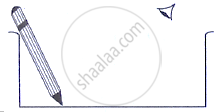(i) What change will be observed in the appearance of the pencil when water is poured into the trough?
(ii) Name the phenomenon which accounts for the above stated observation.
(iii) complete the diagram showing how the student’s eye sees the pencil through water.

Exercise - 4 (C) | Q 8 | Page 91

An object placed in one medium when seen from the other medium, appears to be vertically shifted. Name two factors on which the magnitude of shift depends and state how does it depend on them.

#### Selina solutions for Concise Physics Class 10 ICSE Chapter 4 Refraction of Light at Plane SurfacesExercise Exercise - 4 (C) [Page 92]

Exercise - 4 (C) | Q 1 | Page 92

A small air bubble in a glass block when seen from above appears to be raised because of:

• Refraction of light

• Reflection of light

• Reflection and refraction of light

• None of the above

Exercise - 4 (C) | Q 2 | Page 92

An object in a denser medium when viewed from a rarer medium appears to be raised. The shift is maximum for :

• Red light

• Violet light

• Yellow light

• Green light

#### Selina solutions for Concise Physics Class 10 ICSE Chapter 4 Refraction of Light at Plane SurfacesExercise Exercise - 4 (C) [Page 92]

Exercise - 4 (C) | Q 1 | Page 92

A Water pond appears to be 2.7 m deep. If the refractive index of water is 4/3, find the actual depth of the pond.

Exercise - 4 (C) | Q 2 | Page 92

A coin is places at the bottom of a beaker containing water (refractive index = 4/3) to a depth of 12 cm. By what height the coin appears to be raised when seen from vertically above?

Exercise - 4 (C) | Q 3 | Page 92

A postage stamp kept below a rectangular glass block of refractive index 1.5 when viewed from vertically above it, appears to be raised by 7.0 mm. calculate the thickness of the glass block.

#### Selina solutions for Concise Physics Class 10 ICSE Chapter 4 Refraction of Light at Plane SurfacesExercise Exercise - 4 (D) [Pages 100 - 102]

Exercise - 4 (D) | Q 1 | Page 100

Explain the term critical angle with the aid of a labelled diagram.

Exercise - 4 (D) | Q 2 | Page 100

How is the critical angle related to the refractive index of a medium?

Exercise - 4 (D) | Q 3.1 | Page 100

State the approximate value of the critical angle for glass-air surface

Exercise - 4 (D) | Q 3.2 | Page 100

State the approximate value of the critical angle for water-air surface.

Exercise - 4 (D) | Q 4 | Page 100

What is meant by the statement the critical angle for diamond is 24°?

Exercise - 4 (D) | Q 5 | Page 100

A light ray is incident from a denser medium on the boundary separating it from a rarer mediuma an angle of incident equal to the critical angle, what is the angle of refraction for the ray?

Exercise - 4 (D) | Q 6 | Page 100

Name two factors which affect the critical angle for a given pair of media. State how do the factors affect it.

Exercise - 4 (D) | Q 7 | Page 100

The critical angle for glass-air is 45° for the light of yellow colour. State whether it will be less than, equal to, or more than 45° for (i) ref light, (ii) blue light?

Exercise - 4 (D) | Q 8.1 | Page 100

What is total internal reflection?

Exercise - 4 (D) | Q 8.2 | Page 100

State two conditions necessary for total internal reflection to occur.

Exercise - 4 (D) | Q 8.3 | Page 100

Draw diagram to illustrate the total internal reflection .

Exercise - 4 (D) | Q 9.1 | Page 100

Fill in the blanks to complete the following sentence:

Total internal reflection occurs only when a ray of light passes from a …………….. medium to a ………………. Medium.

Exercise - 4 (D) | Q 9.2 | Page 100

Fill in the blanks to complete the following sentence

critical angle is the angle of ……………. In denser medium for which the angle of ………….. in rarer medium is………………….

Exercise - 4 (D) | Q 10 | Page 100

State whether the following statement is true or false:

If the angle of incidence is greater than the critical angle, light is not refracted at all, when it falls on the surface from a denser medium to a rarer medium.

• True

• False

Exercise - 4 (D) | Q 11 | Page 100

The refractive index of air with respect to glass is expressed as

g μ a=sin i /sin r

(a) Write down a similar expression for a μ g in terms of the angles i and r.
(b) if angle r = 90°, what is the corresponding angle i called?
(c) what is the physical significance of the angle i in part (b)?

Exercise - 4 (D) | Q 12 | Page 100

In following figure , show two rays A and B travelling from water to air. If the critical angle for water- air surface is 48°, complete the ray diagram showing the refracted rays for each. State conditions when the ray will suffer total internal reflection.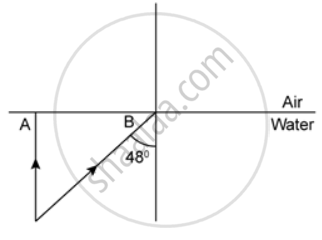Exercise - 4 (D) | Q 13 | Page 101

The given figure shows a point source P inside a water container three rays A,B and C starting from the source P are shown up to the water surface. (a) show in the diagram the path of these rays after striking the water. The critical angle for water-air surface is 48°. (b) Name the phenomenon which the rays A, B and C exhibit.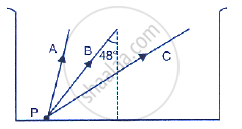Exercise - 4 (D) | Q 14 | Page 101

In the given figure PQ and PR are the two light rays emerging from an object P. The ray PQ is refracted as QS.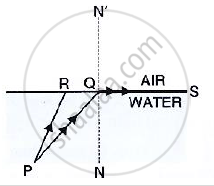(a) state the special name given to the angle of incidence ∠PQN of the ray PQ.
(b) what is the angle of refraction for the refracted ray QS?
(c) name the phenomenon that occurs if the angle of incidence ∠ PQN is increased.
(d) The ray PR suffers partial reflection and refraction on the water-air surface. Give reason. Draw in the diagram the refracted ray for the incident ray PR and hence show the position of image of the object P by the letter P’ when seen vertically from above.

Exercise - 4 (D) | Q 15 | Page 101

The refractive index of glass os 1.5. From a point P inside a glass block, draw rays PA, PB and PC incident on the glass air surface at an angle of incidence 30°, 42° and 60° respectively.
(a) In the diagram show the approximate direction of these rays as they emerge out of the block.
(b) What is the angle of refraction for the ray PB?

(Take sin 42°=2/3

Exercise - 4 (D) | Q 16 | Page 101

A ray of light enters a glass slab ABDC as shown in fig. 4.59 and strikes at the centre O of the circular part AC of the slab. The critical angle of glass is 42°. Complete the path of the ray till it
emerges out from the slab. Mark the angles in the diagram wherever necessary.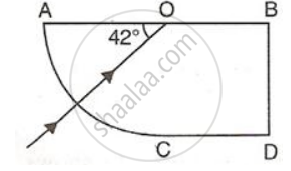Exercise - 4 (D) | Q 17 | Page 101

What is a total reflecting prism? State three actions that it can produce. Draw a diagram to show one action of the total reflecting prism.

Exercise - 4 (D) | Q 18 | Page 101

Show with the help of a diagram how a total reflecting prism can be used to turn a ray of light through 90°. Name one instrument in which such a prism is used.

Exercise - 4 (D) | Q 19 | Page 101

A ray of light OP passes through a right angles prism as shown in the adjacent diagram.
(a) State the angles of incidence at the faces AC and BC.
(b) Name the phenomenon which the ray suffers at the face AC.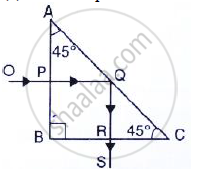Exercise - 4 (D) | Q 20 | Page 101

In following figure , a ray of light PA is incident normally on the hypotenuse of an isoceles right angle prism ABC. (a) Complete the path of the ray PQ till it emerges from the prism. Mark in the diagram the angle wherever necessary. (b) what is the angle of deviation of the ray PQ? (c) Name a device in which this action is used.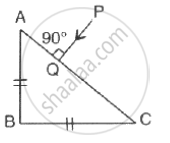Exercise - 4 (D) | Q 21 | Page 101

In given figure a ray of light PQ is incident normally on the face AB of an equilateral glass prism. Complete the ray diagram showing its emergence into air after passing through the prism.
(a) Write the angles of incidence at the faces AB and AC of the prism.
(b) name the phenomenon which the ray of light suffers at the face AB, AC and BC of the prism.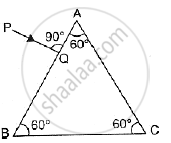Exercise - 4 (D) | Q 22 | Page 102

Draw a neat labelled ray diagram to show the total internal reflection of a ray of light normally incident on one face of a 30°, 90°, 60° prism.

Exercise - 4 (D) | Q 23 | Page 102

Two isosceles right –angles glass prisms are placed near each other as shown in Fig. 4.63. Complete the path of the light ray entering the first prism till it emerges out of the second prism.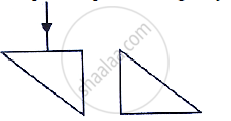Exercise - 4 (D) | Q 24 | Page 102

What device other than a plane mirror can be used to turn a ray of light through 180°? Draw a diagram in support of your answer. Name an instrument in which this device is used.

Exercise - 4 (D) | Q 25 | Page 102

Mention one difference between reflection of light from a plane mirror and total internal reflection of light from a prism.

Exercise - 4 (D) | Q 26 | Page 102

State one advantage of using a total reflecting prism as a reflector in place of plane mirror.

#### Selina solutions for Concise Physics Class 10 ICSE Chapter 4 Refraction of Light at Plane SurfacesExercise Exercise - 4 (D) [Page 102]

Exercise - 4 (D) | Q 1 | Page 102

The critical angle for glass-air interface is :

• 24°

• 48°

• 42°

• 45°

Exercise - 4 (D) | Q 2 | Page 102

A total reflecting right angled isosceles prism can be used to deviate a ray of light through

• 30°

• 60°

• 75°

• 90°

Exercise - 4 (D) | Q 3 | Page 102

A total reflecting equilateral prism can be used to deviate a ray of light through:

• 30°

• 60°

• 75°

• 90°

## Chapter 4: Refraction of Light at Plane Surfaces

Exercise - 4 (A)Exercise - 4 (B)Exercise - 4 (C)Exercise - 4 (D)## Selina solutions for Concise Physics Class 10 ICSE chapter 4 - Refraction of Light at Plane Surfaces

Selina solutions for Concise Physics Class 10 ICSE chapter 4 (Refraction of Light at Plane Surfaces) include all questions with solution and detail explanation. This will clear students doubts about any question and improve application skills while preparing for board exams. The detailed, step-by-step solutions will help you understand the concepts better and clear your confusions, if any. Shaalaa.com has the CISCE Concise Physics Class 10 ICSE solutions in a manner that help students grasp basic concepts better and faster.

Further, we at Shaalaa.com provide such solutions so that students can prepare for written exams. Selina textbook solutions can be a core help for self-study and acts as a perfect self-help guidance for students.

Concepts covered in Concise Physics Class 10 ICSE chapter 4 Refraction of Light at Plane Surfaces are Refraction of Light Through a Glass Block and a Triangular Prism, Total Internal Reflection, Total Internal Reflection - Critical Angle, Applications of Refraction of Light, Refraction of Light Through Plane Surface.

Using Selina Class 10 solutions Refraction of Light at Plane Surfaces exercise by students are an easy way to prepare for the exams, as they involve solutions arranged chapter-wise also page wise. The questions involved in Selina Solutions are important questions that can be asked in the final exam. Maximum students of CISCE Class 10 prefer Selina Textbook Solutions to score more in exam.

Get the free view of chapter 4 Refraction of Light at Plane Surfaces Class 10 extra questions for Concise Physics Class 10 ICSE and can use Shaalaa.com to keep it handy for your exam preparation

S